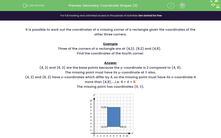# Geometry: Coordinate Shapes (3)

In this worksheet, students work out the coordinates of the missing corner of a rectangle, given the other three corners.Key stage:  KS 2

Curriculum topic:   Geometry: Position and Direction

Curriculum subtopic:   Know, Describe and Present Shapes

Popular topics:   Geometry worksheets

Difficulty level:#### Worksheet Overview

It is possible to work out the coordinates of a missing corner of a rectangle given the coordinates of the other three corners.

Example

Three of the corners of a rectangle are at (4,2), (8,2) and (4,8).

Find the coordinates of the fourth corner.

(4, 2) and (8, 2) are the base points because the y-coordinate is 2 compared to (4, 8).

The missing point must have its y-coordinate at 8 also.

(4, 2) and (8, 2) have x-coordinates which differ by 4, so the missing point must have its x-coordinate 4 more than (4,8).....i.e. 4 + 4 = 8.

The missing point has coordinates (8, 8).### What is EdPlace?

We're your National Curriculum aligned online education content provider helping each child succeed in English, maths and science from year 1 to GCSE. With an EdPlace account you’ll be able to track and measure progress, helping each child achieve their best. We build confidence and attainment by personalising each child’s learning at a level that suits them.

Get started